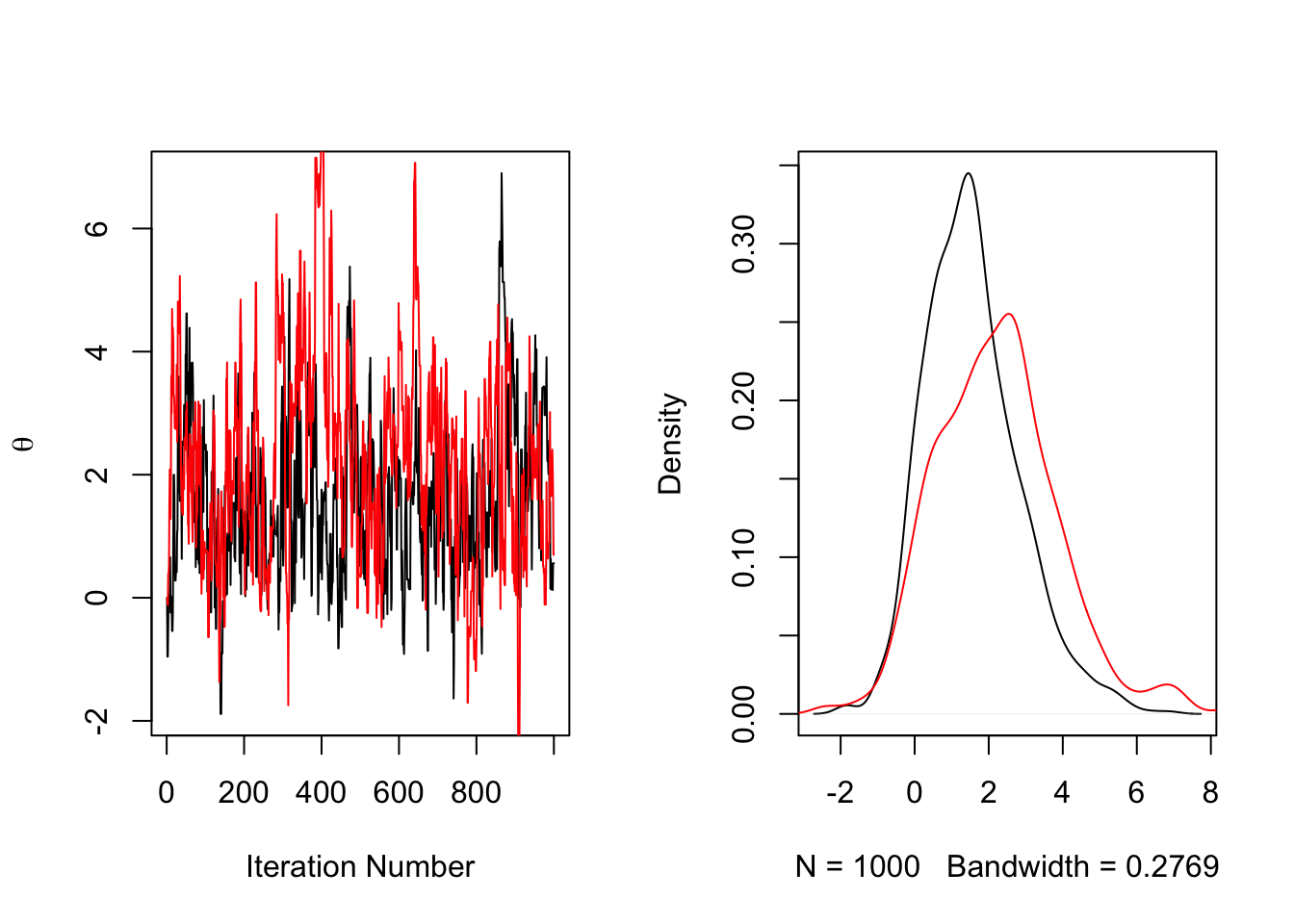## In This Lecture:

1. Git (and how to access the course GitHub repo)
2. RStudio (and how to use Git within RStudio)
3. R Markdown (and how to make it run to play with syntax during class)

## Git Clients

• If you use a Mac/Linux machine, you already have a client as part of the OS.
• If you use Windows, you will have to download and install a client to use Git

## Using Git with R Studio

• Git makes our life easy when used with RStudio as we can use it to keep a current copy of our course notes available
• We will use Git to download and update our course materials in RStudio
• Do do this:
1. Open RStudio
2. At the menu on the top, go to File…New Project
3. Select “Version Control” on the “Create Project” window
4. Select “Git” on the “Create Project from Version Control” window
5. On the “Clone Git Repository” window input:
• Repository URL: https://github.com/jonathantemplin/BayesianPsychometricModeling
• Project directory name: Choose a directory name for the course materials (such as “BPM Git Repo”)
• NOTE: The master branch has an up-to-date R project file (.Rproj) that will have all files included.
• Create project as a subdirectory of: Choose a location for your files to reside within on your local machine

## Using RStudio

• Next I will demonstrate RStudio for you using the contents of last week’s R script file
• Also, to really unlock RStudio’s full potential, familiarize yourself with its keyboard shortcuts:
• In the top menu, go to Tools…Keyboard Shortcuts Help

## Using R Markdown

• Last week was the exception in that I did not provide course materials in R Markdown…today that changes

• R Markdown is a form of the Markdown language (https://en.wikipedia.org/wiki/Markdown)

• Markdown was the counter to HTML (Hypertext Markup Language)…but is a markup language that is very easy to use
• You can find Markdown nearly everywhere these days (see your Notes/OneNote application)
• Markdown makes writing very simple:

• It works nearly everywhere (files are simple text)
• It can incorporate more complicated markup languages, such as LaTex: $$P \left(\theta|Y \right) \propto P \left(Y|\theta \right) P \left( \theta \right)$$
• When you do need a type of document, you can then use any number of programs to make it look nice:

• R Markdown allows you to embed R script within the document, providing syntax snippets and output directly to your final document format

• You can find lots of helpful tips on R Markdown on some of these sites:

## R Markdown Example

• Recalling last week’s R script for theta, below is how to embed it in R Markdown
• Note: I’ve changed the number of iterations to something very small to make it run fast
irtItemProb = function(a, b, c=0, theta){
prob = c + (1-c) * exp(a*(theta-b))/(1+exp(a*(theta-b)))
return(prob)
}

trueTheta = 0
nItems = 5
nItems = 5
bRange = c(-2,2)
aRange = c(1,2)
bSE = 1
aSE = 1
nSamples = 1000

# draw mean values of a, b
a = runif(n = nItems, min = aRange, max = aRange)
b = runif(n = nItems, min = bRange, max = bRange)

# draw items
itemResponses = rbinom(n = nItems, size = 1, prob = irtItemProb(a = a, b = b, theta = 1))
thetaChain = list(rep(NA, nSamples), rep(NA, nSamples))

# initialize theta values
curTheta = trueTheta
curThetaRand = trueTheta
for (iteration in 1:nSamples){

# draw item parameters (if random)
iterA = rnorm(n = nItems, mean = a, sd = aSE)
iterB = rnorm(n = nItems, mean = b, sd = bSE)

# calculate current likelihood of the data | theta
curLogLike = sum(dbinom(x = itemResponses, size = 1, prob = irtItemProb(a = a, b = b, theta = curTheta), log = TRUE))
curLogLikeRand = sum(dbinom(x = itemResponses, size = 1, prob = irtItemProb(a = iterA, b = iterB, theta = curThetaRand), log = TRUE))

# draw new theta value
propTheta = rnorm(n = 1, mean = curTheta, sd = 1)
propThetaRand = rnorm(n = 1, mean = curThetaRand, sd = 1)

# calculate proposed likelihood of the data | theta
propLogLike = sum(dbinom(x = itemResponses, size = 1, prob = irtItemProb(a = a, b = b, theta = propTheta), log = TRUE))
propLogLikeRand = sum(dbinom(x = itemResponses, size = 1, prob = irtItemProb(a = iterA, b = iterB, theta = propThetaRand), log = TRUE))

# do MH:
if (log(runif(n = 1)) < (propLogLike-curLogLike)){
# accept
curTheta = propTheta
}

# do MH:
if (log(runif(n = 1)) < (propLogLikeRand-curLogLikeRand)){
# accept
curThetaRand = propThetaRand
}

thetaChain[][iteration] = curTheta
thetaChain[][iteration] = curThetaRand
}

par(mfrow = c(1,2))

plot(thetaChain[], type="l", ylab = expression(theta), xlab = "Iteration Number")
lines(thetaChain[], type="l", col = 2)
plot(density(thetaChain[]), col = 1, main="")
lines(density(thetaChain[]), col = 2)par(mfrow = c(3,2))
plot(thetaChain[], type="l", ylab = expression(theta), xlab = "Iteration Number")
plot(thetaChain[], type="l", ylab = expression(theta), xlab = "Iteration Number", col =2)
plot(density(thetaChain[]), col = 1, main="")
plot(density(thetaChain[]), col = 2, main="")
plot(thetaChain[], type="l", ylab = expression(theta), xlab = "Iteration Number")
lines(thetaChain[], type="l", col = 2)
plot(density(thetaChain[]), col = 1, main="")
lines(density(thetaChain[]), col = 2)